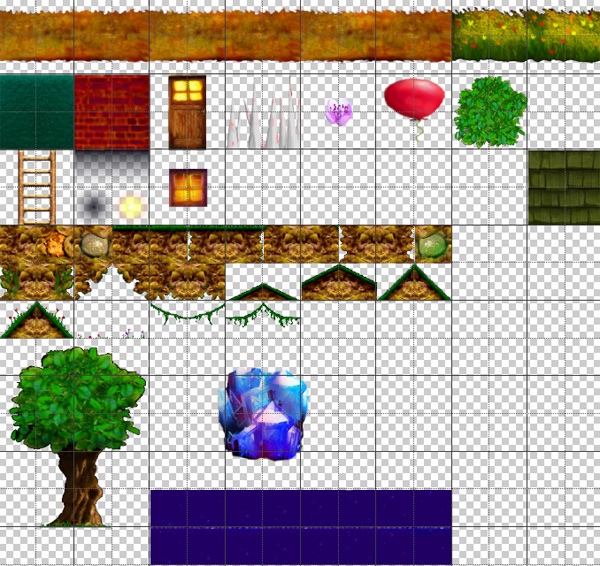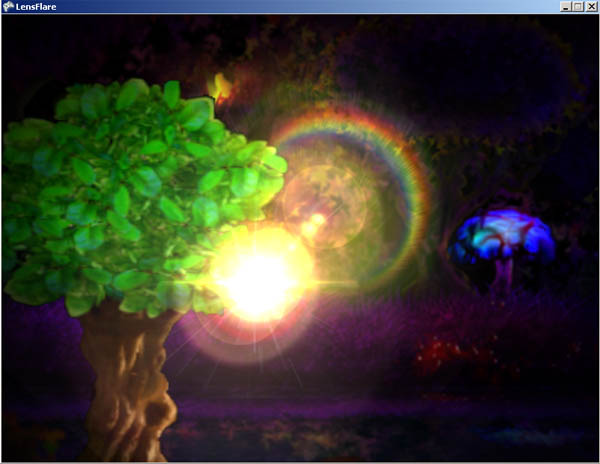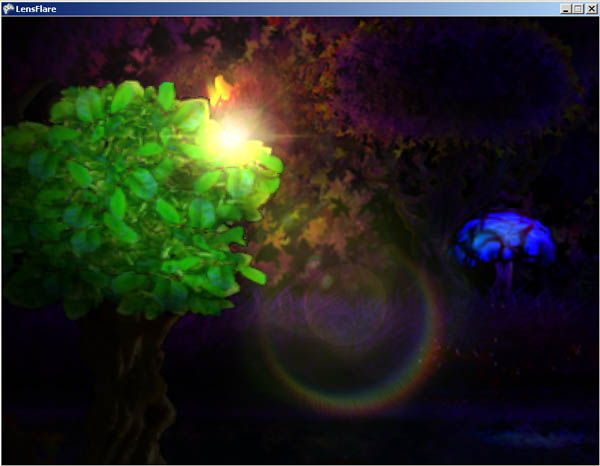## XNA (or Monogame) TUTORIAL: Obstructing Light Effects (Foreground Obstructions)

GOAL: Continuing from the previous tutorial (Lens Flares and Lighting), we will add images which will block the light source but we want the intense part of the light to try to bleed over the edge of the object before fading from sight. I'm using the idea of representing the center of the light as a pixel point so if the radius of the light image begins to fall behind an object, it starts to scale down the intensity of the light and hides it completely once the center is hiddin.

To make it more interesting we will use a scaled forground image (a tree) which will rock slowly in the wind. This should block out the light effect when we move the mouse behind it.

Step 1) You will need to re-Open the project you created in the Lens Flare tutorial. Add a tileSheet of some sort with an image (like a tree) to the Content Pipeline. This is what the tileSheet I made for this project looks like:Step 2) We'll add the variables and functionality to make the tree sway. At the top of main.cs (or game1.cs), add these:

Code:
```#region N E W  V A R S ----------
Texture2D tileSheet;
Rectangle treeRect;
float tree_wave = 0f, tree_timer = 0f;
#endregion```

Step 3) We need to load the tileSheet, and set the source rectangle for the tree:
Code:
```protected override void LoadContent()
{

treeRect = new Rectangle(2, 578, 251, 318);
}```
Step 4) Let's sway the tree back and forth slowly in the Update:
Code:
```        //added:
//make the tree rock back and forth slowly...
tree_timer += 0.014f; //rock speed
tree_wave = (float)Math.Sin(tree_timer)/20.0f; //divide by 20 to reduce angle of rocking
base.Update(gameTime);
}        ```
Step 5) In the Draw method, where it says "//Draw all normal scene stuff here" we will draw the tree rotating it by creating a rotation angle using SIN of tree_wave(as calculated in Update) and using a scale of 1.8(big and in foreground) and I manually set the origin(center of rotation) near the middle bottom of the tree:
Code:
```        spriteBatch.Draw(fxTexture, mosV, light, lightColor, 0f, light_origin, 2f, SpriteEffects.None, 0f);
spriteBatch.Draw(tileSheet, new Vector2(150,610), treeRect, Color.White, (float)Math.Sin(tree_wave), new Vector2(128f,304f), 1.8f, SpriteEffects.None, 0f);
//Draw all normal scene stuff here
//--------------------------------
spriteBatch.End();```

Now if you run it you should have a swaying tree which we'll using as the obstruction. :NOTE: There are a few different ways to test for occlusion(and occlusion amount).

Step 6) To check if the light is hidden behind the tree (or partly hidden), we'll draw the foreground seperately at a different depth in the depth buffer. Then we'll call an occlusionQuery to test how many pixels are covered. But... before we do that we must also disable writing transparent pixels to the depth buffer (otherwise the entire rectangle of the tree will block out the light)... to do this we set up an alpha test to be passed into Begin().
The surface area of the light we want test for should actually be somewhat smaller than the light sprite. Let's set some variables in main.cs(or game1.cs):
Code:
```        float tree_wave = 0f, tree_timer = 0f;
const float    testSize = 80, testArea = testSize * testSize;
bool           lightVisible;
OcclusionQuery occlusionTest;
float          occlusionAlpha;
bool           occlusionTestActive;
AlphaTestEffect alpha_test;```

testArea will be the maximum number of pixels from the light that could be visible.
occlusionAlpha will control how transparent the lights are (based on percent of area visible)
alpha_test is used to tell the gpu not to draw transparent pixels to the depth buffer (or at all)
occlusionTestActive - makes sure it has at least been started once before we do anything silly... ;p

Step 7) In the constructor of main.cs(or game1.cs), change it so we use depth16 like so:

Code:
```//--------------------CHANGED------------------------------
graphics = new GraphicsDeviceManager(this) {
PreferredBackBufferWidth = SCREENWIDTH, PreferredBackBufferHeight = SCREENHEIGHT, IsFullScreen = FULLSCREEN,
PreferredDepthStencilFormat = DepthFormat.Depth16
};
//---------------------------------------------------------```

Step 8) In Initialize, we need to create an instance of OcclusionQuery and AlphaTestEffect. (note the projection matrix is set up to be like the one used in spritebatch).
Code:
```//Added-----------------
occlusionTest = new OcclusionQuery(GraphicsDevice);

// Need alpha test when drawing foreground, otherwise transparent pixels will have depth and be considered occluding the light
alpha_test = new AlphaTestEffect(GraphicsDevice); //alpha_test.VertexColorEnabled = true;//alpha_test.AlphaFunction = CompareFunction.; //alpha_test.ReferenceAlpha =;
alpha_test.Projection = Matrix.CreateTranslation(-0.5f, -0.5f, 0) * Matrix.CreateOrthographicOffCenter(0, SCREENWIDTH, SCREENHEIGHT, 0, 0, 1);

base.Initialize();```
Step 9) Between Update and Draw, we'll add a method to test for occluded pixels. It will return if the light is out of view or if the previous occlusion test (happening on the GPU) isn't done yet (as the CPU might be ready but not the GPU yet) -- otherwise if the previous test has finished, we can calculate the alpha based on how many pixels were visible. So we calculate the alpha(or percentage) of visibility by dividing the count by the total (our testArea const).
To do the actual occlusion test, we draw the light bit to test pixel visibility for in the occlusion test Begin,End and we do it in the middle of the scene(0.5f).. If you were doing a distant sun or something, it would be more like 1.0f
Code:
```//--------------------------------------
#region O C C L U S I O N   S O L V E R
//--------------------------------------
void OcclusionSolver()
{
// Don't draw any flares if the light isn't visible (and return if not on screen)
if ((mosV.X < -80 ) || (mosV.X > SCREENWIDTH+80) || (mosV.Y < -80) || (mosV.Y > SCREENHEIGHT+80)) { lightVisible = false; return; } else lightVisible = true;
if (occlusionTestActive)
{
if (!occlusionTest.IsComplete) return; // don't continue if the previous query hasn't finished (happening on gpu)
occlusionAlpha = Math.Min(occlusionTest.PixelCount / testArea, 1)*2; // Use pixel count to calculate percentage of visible light (*2 just to boost it up)
}
occlusionTest.Begin();
spriteBatch.Begin(SpriteSortMode.BackToFront, BlendState.NonPremultiplied, SamplerState.PointClamp, DepthStencilState.Default, RasterizerState.CullNone, null);
spriteBatch.Draw(fxTexture, new Rectangle((int)mosV.X,(int)mosV.Y,(int)testSize,(int)testSize), light, new Color(255,255,255,1), 0f, light_origin, SpriteEffects.None, 0.5f);
spriteBatch.End();
occlusionTest.End();
occlusionTestActive = true;
}
#endregion```

Note: I multiplied the final alpha by 2 to amplify the result a bit just because I thought it looked a bit better.. also note that we don't want to return or calculate occlusionAlpha unless we've started the occlusion test at least once - thus: occlusionTestActive = true

Step 10) This is a big step. We need to modify our draw method a lot. In the part wher we draw our regular scene stuff, we need to inlude alpha_test in spriteBatch.Begin to ensure we don't draw unwanted pixels to the depth buffer.
NOTE: Something strange happens with the depth values we need to use due to alpha_test. It seems we need to use negative values for the depths instead of positive... I'm not actually sure why yet.... but it works... ;p
After drawing the regular scene stuff, we make a call to the occlusion solver, and then blend in the lights as before.

Now though, we multiply the final alpha (occlusionAlpha) by the colors used in rendering the lens flares. This will give us a nice fade as we move the light under an occluder.

Here's the final Draw method:

Code:
```    protected override void Draw(GameTime gameTime)
{
// 1) D R A W   L I G H T S   O N   T A R G E T-------------------------
GraphicsDevice.SetRenderTarget(lightsTarget);
GraphicsDevice.Clear(new Color(40,50,60));    //Could use Black - I'm using gray so there's some ambient lighting
//(note need to divide coordinates by 4 because the target I'm using is 4 times smaller)
//place a BLUE light in the mushroom:
spriteBatch.End();
//----------------------------------------------------------------------

// 2) D R A W   S C E N E ----------------------------------------------
GraphicsDevice.SetRenderTarget(null); //use normal backbuffer to render now
GraphicsDevice.Clear(ClearOptions.Target | ClearOptions.DepthBuffer, Color.Black, 1.0f, 0);
spriteBatch.Begin(SpriteSortMode.BackToFront, BlendState.NonPremultiplied, SamplerState.PointClamp, DepthStencilState.Default, RasterizerState.CullNone, alpha_test);

spriteBatch.Draw(fxTexture, backDest, background, Color.White, 0f, Vector2.Zero, SpriteEffects.None,-0.9f);
//Draw all background and main scene stuff here:
//::::::::::::::::::::::::::::::::::::::::::::::

//Draw all foreground (occluding/obstructing) stuff here
spriteBatch.Draw(tileSheet, new Vector2(150,610), treeRect, Color.White, (float)Math.Sin(tree_wave), new Vector2(128f,304f), 1.8f, SpriteEffects.None, -0.1f);

spriteBatch.End();
OcclusionSolver(); // O C C L U S I O N  T E S T
//----------------------------------------------------------------------

// 3) B L E N D   I N   L I G H T I N G   F R O M   T A R G E T---------------
//Now using the blendstate we created, add the lights made on the lights target
spriteBatch.Begin(SpriteSortMode.BackToFront, blendState, null, null, null);
spriteBatch.Draw(lightsTarget, new Rectangle(0,0,SCREENWIDTH,SCREENHEIGHT), Color.White);
spriteBatch.End();
//----------------------------------------------------------------------------

// 4) D R A W   L E N S   F L A R E   W I T H   A L P H A   F R O M   O C C L U S I O N   T E S T-----------------
//draw lens flare effect over everything (using Additive blending):
if ((occlusionAlpha > 0)&&(lightVisible))
{
spriteBatch.Draw(fxTexture, mosV, light, lightColor*occlusionAlpha, 0f, light_origin, 2f, SpriteEffects.None, 0f);
Color final_color;
int i = 0;
do
{
final_color = flares[i].color*occlusionAlpha;
spriteBatch.Draw(fxTexture, flares[i].pos, flares[i].rect, final_color, flares[i].rot, flares[i].origin, flares[i].scale + light_scale / 10, SpriteEffects.None, 0);
i++;
} while (i < num_flares);
}
spriteBatch.End();
//----------------------------------------------------------------------------------------------------------------

old_kb = kb; old_mos = mos;
base.Draw(gameTime);
}
#endregion
}```

Here you can see the light and flares are starting to fade a bit due to being partly obstructed by the tree:Project Source Code